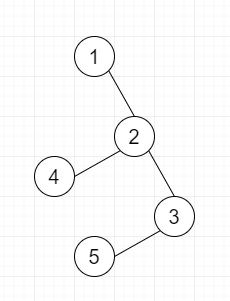# Find the number of distinct pairs of vertices which have a distance of exactly k in a tree in Python

Suppose we have an integer k and also have a tree with n nodes, we have to count the number of distinct pairs of vertices which have a exact k distance.

So, if the input is like k = 2then the output will be 4

To solve this, we will follow these steps −

• N := 5005

• graph := adjacency list of size N

• vertex_count := a 2d matrix of size 505 x 5005

• res := 0

• Define a function insert_edge() . This will take x, y

• insert y at the end of graph[x]

• insert x at the end of graph[y]

• Define a function dfs() . This will take v, parent

• vertex_count[v, 0] := 1

• for each i in graph[v], do

• if i is not same as parent, then

• dfs(i, v)

• for j in range 1 to k + 1, do

• res := res + vertex_count[i, j - 1] * vertex_count[v, k - j]

• for j in range 1 to k + 1, do

• vertex_count[v, j] := vertex_count[v, j] + vertex_count[i, j - 1]

## Example

Let us see the following implementation to get better understanding −

Live Demo

N = 5005
graph = [[] for i in range(N)]
vertex_count = [[0 for i in range(505)] for i in range(N)]
res = 0
def insert_edge(x, y):
graph[x].append(y)
graph[y].append(x)
def dfs(v, parent):
global res
vertex_count[v] = 1
for i in graph[v]:
if (i != parent):
dfs(i, v)
for j in range(1, k + 1):
res += vertex_count[i][j - 1] * vertex_count[v][k - j]
for j in range(1, k + 1):
vertex_count[v][j] += vertex_count[i][j - 1]
k = 2
insert_edge(1, 2)
insert_edge(2, 3)
insert_edge(3, 4)
insert_edge(2, 5)
dfs(1, 0)
print(res)

## Input

k = 2
insert_edge(1, 2)
insert_edge(2, 3)
insert_edge(3, 4)
insert_edge(2, 5)

## Output

4